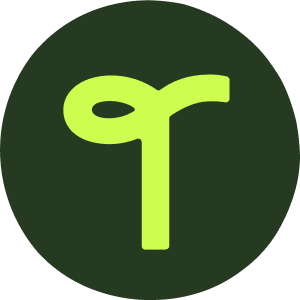teaching resource

Number Hunt Description Cards

A set of 32 task cards that have students use their knowledge of place value to find specific numbers.

This teaching resource is a great way to practice students’ place value knowledge through physical activity.

This set of 32 task cards prompts students to find numbers that fit a specific description, all while performing some type of physical movement. Simply spread out a set of numbers you feel are appropriate for your students and read a description card. While performing the action given on the card, students must simultaneously find a number that matches the description. Once everyone has found a spot, have each child read their number to the class.

These task cards are leveled for your convenience. The first 16 green cards can be used with the numbers 0–20, while the remaining 16 yellow cards are meant to be used with numbers 0–120.

This resource works great with the 0-120 Number and Word Flashcards – Circles.

0-120 Number and Word Flashcards - Circles

Use these flashcards when learning about numbers and words.31 pagesGrades: K - 2

Curriculum

• CCSS.MATH.CONTENT.K.CC.A.3

Write numbers from 0 to 20. Represent a number of objects with a written numeral 0-20 (with 0 representing a count of no objects).

• CCSS.MATH.CONTENT.K.CC.C.7

Compare two numbers between 1 and 10 presented as written numerals.

• CCSS.MATH.CONTENT.1.NBT.B.2

Understand that the two digits of a two-digit number represent amounts of tens and ones. Understand the following as special cases:

• CCSS.MATH.CONTENT.1.NBT.B.2.C

The numbers 10, 20, 30, 40, 50, 60, 70, 80, 90 refer to one, two, three, four, five, six, seven, eight, or nine tens (and 0 ones).

• CCSS.MATH.CONTENT.1.NBT.B.3

Compare two two-digit numbers based on meanings of the tens and ones digits, recording the results of comparisons with the symbols >, =, and

• CCSS.MATH.CONTENT.2.NBT.A.1

Understand that the three digits of a three-digit number represent amounts of hundreds, tens, and ones; e.g., 706 equals 7 hundreds, 0 tens, and 6 ones. Understand the following as special cases:

• CCSS.MATH.CONTENT.2.NBT.A.3

Read and write numbers to 1000 using base-ten numerals, number names, and expanded form.

• CCSS.MATH.CONTENT.2.NBT.A.4

Compare two three-digit numbers based on meanings of the hundreds, tens, and ones digits, using >, =, and < symbols to record the results of comparisons.